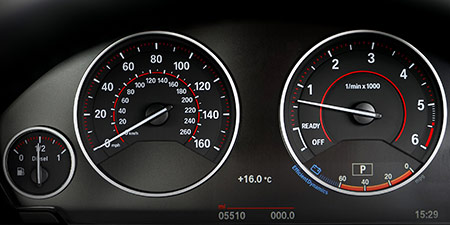# Kilometers per hour (km/h) to Kilometers per second (km/s)

Units of speed ﹣ Converter Kilometers per hour to Kilometers per second

Here you can convert the Speed unit Kilometers per hour into the unit Kilometers per second and vice versa you can convert Kilometers per second into Kilometers per hour. By clicking the "Swap units" icon, you will always obtain the desired conversion in the calculation result, i.e., km/h to km/s or km/s to km/h. With the following calculator you can also calculate any other Speed unit.

## Info about "Kilometers per hour"

Kilometers per hour is used as a standard unit in transportation, therefore it is used in traffic or to measure the travel speed of vehicles. For example, a commercial aircraft flies at a speed of 1,000 km/h then it covers a distance of 1,000 kilometers per hour.

The unit is defined as follows: An object moving at 1 "km/h" per hour will cover one kilometer. "km/h" is approved to use in the international system of units (SI).

## Info about "Kilometers per second"

Kilometer per second is part of the metric system and it is derived from the standard unit of speed, meter per second (m/s). This is because both meters and seconds are defined as the basic units for length and time respectively.

This unit is defined as follows: An object moving at "1 km/s" per second will cover one kilometer. "km/s" is approved to use in the international system of units (SI).

## Basis for conversion Kilometers per hour (km/h) to Kilometers per second (km/s) and vice versa

The abbreviation for the "Speed unit Kilometers per hour" is km/h. The abbreviation for the "Speed unit Kilometers per second" is km/s.

## Formula for the conversion of Kilometers per hour (km/h) to Kilometers per second (km/s) and vice versa

The calculation from Kilometers per hour to Kilometers per second shall be made using the following conversion formula:

Conversion formula Kilometers per hour to Kilometers per second

Determine the number of Kilometers per second from Kilometers per hour

Kilometers per hour × 0.00027777777777778

## Formula for the conversion of Kilometers per second (km/s) to Kilometers per hour (km/h)

The calculation from Kilometers per second to Kilometers per hour shall be made using the following conversion formula:

Conversion formula Kilometers per second to Kilometers per hour

Determine the number of Kilometers per hour from Kilometers per second

Kilometers per second × 3600

## Overview table : How many Kilometers per hour are in a Kilometers per second ?

Kilometers per hour km/h ⇒ Kilometers per second km/s
0.04 km/h  are  0.00001 km/s
0.05 km/h  are  0.00001 km/s
0.06 km/h  are  0.00001 km/s
0.07 km/h  are  0.00001 km/s
0.08 km/h  are  0.00002 km/s
0.09 km/h  are  0.00002 km/s
0.10 km/h  are  0.00002 km/s
0.20 km/h  are  0.00005 km/s
0.30 km/h  are  0.00008 km/s
0.40 km/h  are  0.00011 km/s
0.50 km/h  are  0.00013 km/s
0.60 km/h  are  0.00016 km/s
0.70 km/h  are  0.00019 km/s
0.80 km/h  are  0.00022 km/s
0.90 km/h  are  0.00025 km/s
1 km/h  corresponds to  0.00027 km/s
2 km/h  are  0.00055 km/s
3 km/h  are  0.00083 km/s
4 km/h  are  0.00111 km/s
5 km/h  are  0.00138 km/s
6 km/h  are  0.00166 km/s
7 km/h  are  0.00194 km/s
8 km/h  are  0.00222 km/s
9 km/h  are  0.00250 km/s
10 km/h  are  0.00277 km/s
20 km/h  are  0.00555 km/s
30 km/h  are  0.00833 km/s
40 km/h  are  0.01111 km/s
50 km/h  are  0.01388 km/s
60 km/h  are  0.01666 km/s
70 km/h  are  0.01944 km/s
80 km/h  are  0.02222 km/s
90 km/h  are  0.02500 km/s
100 km/h  are  0.02777 km/s
200 km/h  are  0.05555 km/s
300 km/h  are  0.08333 km/s
400 km/h  are  0.11111 km/s
500 km/h  are  0.13888 km/s
600 km/h  are  0.16666 km/s
700 km/h  are  0.19444 km/s
800 km/h  are  0.22222 km/s
900 km/h  are  0.25000 km/s
1 000 km/h  are  0.27777 km/s

## Overview table : How many Kilometers per second are in a Kilometers per hour ?

Kilometers per second km/s ⇒ Kilometers per hour km/h
0.01 km/s  are  36 km/h
0.02 km/s  are  72 km/h
0.03 km/s  are  108 km/h
0.04 km/s  are  144 km/h
0.05 km/s  are  180 km/h
0.06 km/s  are  216 km/h
0.07 km/s  are  252 km/h
0.08 km/s  are  288 km/h
0.09 km/s  are  324 km/h
0.10 km/s  are  360 km/h
0.20 km/s  are  720 km/h
0.30 km/s  are  1 080 km/h
0.40 km/s  are  1 440 km/h
0.50 km/s  are  1 800 km/h
0.60 km/s  are  2 160 km/h
0.70 km/s  are  2 520 km/h
0.80 km/s  are  2 880 km/h
0.90 km/s  are  3 240 km/h
1 km/s  corresponds to  3 600 km/h
2 km/s  are  7 200 km/h
3 km/s  are  10 800 km/h
4 km/s  are  14 400 km/h
5 km/s  are  18 000 km/h
6 km/s  are  21 600 km/h
7 km/s  are  25 200 km/h
8 km/s  are  28 800 km/h
9 km/s  are  32 400 km/h
10 km/s  are  36 000 km/h
20 km/s  are  72 000 km/h
30 km/s  are  108 000 km/h
40 km/s  are  144 000 km/h
50 km/s  are  180 000 km/h
60 km/s  are  216 000 km/h
70 km/s  are  252 000 km/h
80 km/s  are  288 000 km/h
90 km/s  are  324 000 km/h
100 km/s  are  360 000 km/h
200 km/s  are  720 000 km/h
300 km/s  are  1 080 000 km/h
400 km/s  are  1 440 000 km/h
500 km/s  are  1 800 000 km/h
600 km/s  are  2 160 000 km/h
700 km/s  are  2 520 000 km/h
800 km/s  are  2 880 000 km/h
900 km/s  are  3 240 000 km/h
1 000 km/s  are  3 600 000 km/h

## Source information

As source for the information in the 'Units of speed' category, we have used in particular:

## Last update on February 19, 2023

The pages of the 'Units of speed' category were last editorially reviewed by Stefan Banse on February 19, 2023. They all correspond to the current status.

### Previous changes on October 9, 2021

• November 9, 2020: Publication of the Speed converter
• Editorial revision of all texts in this category